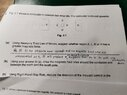# Identifying which region of a conductor experiences the greater force

• ellieee
In summary, the third option has the greatest magnetic force because it has the strongest current loop.f

#### ellieee

Homework Statement
using newton's third law of motion, suggest whether region K,L,M, or N has a greater magnetic force
Relevant Equations
nil
I feel that its M because as the conductor moves upwards, towards K, an equal but opposite force will be produced at M to pull the conductor downwards, but answer is K.

#### Attachments

•16253163523873644178136613189803.jpg
55.6 KB · Views: 62
Homework Statement:: using Newton's third law of motion, suggest whether region K,L,M, or N has a greater magnetic force
Relevant Equations:: nil

I feel that its M because as the conductor moves upwards, towards K, an equal but opposite force will be produced at M to pull the conductor downwards, but answer is K.
Hi @ellieee. I think there are two faults in the question.

1) The shape/configuration of the conductor is unclear. The circle shown could be:
- a cross-section through a single straight wire;
- a loop of thin wire;
- a cross-section through a wire which is part of a bigger loop (not shown).
I’ll assume the 3rd option is correct.

2) The question asks where the ‘magnetic force’ is greatest; but I think it means magnetic field (or the question doesn’t make sense).

As the conductor moves upwards, I agree there is a downwards force it. I think the easiest way to explain the direction of this force is to use Lenz’s law. You can think of the force as arising due to the interaction of the magnet’s field and the field from the induced current.

I suggest you study this: https://physicsmax.com/wp-content/uploads/2014/08/1533.jpg
Remember the stronger a magnetic field, the closer-together are the field lines. Then you should be able to complete the questions.

- a cross-section through a single straight wire;
- a loop of thin wire;
- a cross-section through a wire which is part of a bigger loop (not shown).
how are these 3 options different?

how are these 3 options different?
The first option is a single straight rod perpendicular to the plane of the diagram. (Which means there is no circuit-path for current to flow through the rod.)

The second option is a ring (like you wear on your finger) lying in the plane of the diagram.

The third option is a complete loop (e.g. a square loop) perpendicular to the plane of the the diagram but with only one section of the loop actually inside the field, a bit like this:
https://study.com/cimages/multimages/16/es24796088383046639450301.png

I'm pretty sure they mean the third option, but it's only an educated guess.

Last edited: